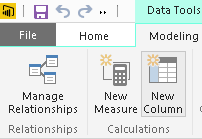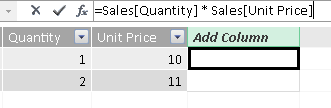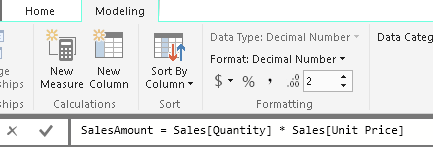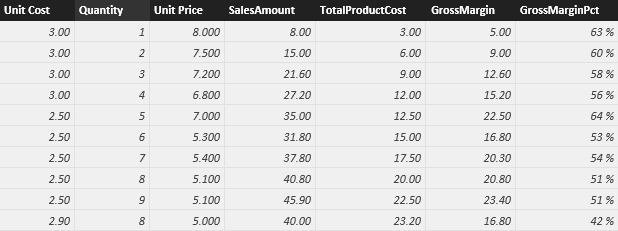Calculated columns

When you create a data model in Power Pivot for Excel, Analysis Services Tabular, or Power BI Desktop, you can extend a table by creating new columns. The content of the columns is defined by a DAX expression evaluated row by row. The user interface is different depending on the tools you use. Excel and Analysis Services require you to write the expression in the formula textbox when you select the last column on the right – “Add Column”. You can rename the new column before or after defining the expression by right-clicking the new column and selecting the Rename Column menu item. As you see in the following picture, the DAX formula you write does not contain the column name and starts with the assignment symbol (=).In Power BI Desktop, you have a different user interface. You have to click the New Column button in order to create a new column.The new column name is part of the formula you write in the formula textbox.The user interface allows you to simply define a new column, but we talk about calculated column to make a distinction between native columns (those read from the data source or evaluated by a query written in Power Query or Power BI) and calculated columns (those created extending a table in the data model).

A calculated column is just like any other column in a table and you can use it in any part of a report. You can also use a calculated column to define a relationship if needed. The DAX expression defined for a calculated column operates in the context of the current row across that table. Any reference to a column returns the value of that column for the current row. You cannot directly access the values of other rows.

One important concept that you need to remember about calculated columns is that they are computed during the database processing and then stored in the model. This might seem strange if you are accustomed to SQL-computed columns – not persisted – which are computed at query time and do not use memory. In data models for DAX, however, all calculated columns occupy space in memory and are computed during table processing.

This behavior is helpful whenever you create very complex calculated columns. The time required to compute them is always process time and not query time, resulting in a better user experience. Nevertheless, you must always remember that a calculated column uses precious RAM. If, for example, you have a complex formula for a calculated column, you might be tempted to separate the steps of computation in different intermediate columns. Although this technique is useful during project development, it is a bad habit in production because each intermediate calculation is stored in RAM and wastes precious space.

For convenience, when writing a formula for a calculated column in an article or in a book, we use the following convention:

TableName[ColumnName] = <DAX expression for calculated column>

This syntax does not correspond to what you input in the user interface, but makes it easy to concisely write the name of the calculated column, the table it belongs to, and its DAX expression. Depending on the tool you use, you have to omit the table name or both table name and column name in the formula you enter in the user interface. For example, consider the following expression in an article:

Sales[GrossMargin] = Sales[SalesAmount] - Sales[TotalProductCost]

In Excel and Analysis Services, you would go in the Sales table and add in a new column the following formula:

= Sales[SalesAmount] - Sales[TotalProductCost]

In Power BI Desktop, you would go in the Sales table, click the New Column button, and type the following formula:

GrossMargin = Sales[SalesAmount] - Sales[TotalProductCost]

Measures

There is another way of defining calculations in a DAX model, useful whenever you do not want to compute values for each row but, rather, you want to aggregate values from many rows in a table. These calculations are measures. This is the same name used in the user interface, with the exception of Excel 2013, which uses the term “calculated field” instead of “measures”. Excel 2016 reverted back to “measures”, which is the term used in DAX and originally used in Power Pivot for Excel 2010, too.

In the previous example you learned how to define the GrossMargin column in the Sales table to compute the gross margin amount. However, what happens if you want to show the gross margin as a percentage of the sales amount? You could create a calculated column with the following formula:

Sales[GrossMarginPct] = DIVIDE ( Sales[GrossMargin], Sales[SalesAmount] )

This formula computes the right value at the row level, as you can see in the following picture.Nevertheless, when computing the aggregate value of a percentage, you cannot rely on calculated columns. Instead you need to compute the aggregate value as the sum of gross margin divided by the sum of sales amount. Therefore, in this case, you need to compute the ratio on the aggregates – you cannot use an aggregation of calculated columns. In other words, you compute the ratio of the sums, not the sum of the ratio.

You cannot use a calculated column for this operation. If you need to operate on aggregate values instead of on a row-by-row basis, you must create measures.

For convenience, when writing a formula for a measure in an article or in a book, we use the convention:

TableName[MeasureName] := <DAX expression for measure>

This syntax simplifies the definition of the name of the measure, of the table it belongs to, and of its DAX expression. Depending on the tool you use, you have to use a different syntax when entering the formula in the user interface. For example, consider the correct implementation for the GrossMarginPct defined as a measure:

Sales[Gross Margin %] := DIVIDE ( SUM ( Sales[GrossMargin] ),  SUM (Sales[SalesAmount] ) )

In Excel and Analysis Services, you would go in the measure grid of the Sales table and type the following text in an empty cell:

Gross Margin % := DIVIDE ( SUM ( Sales[GrossMargin] ), SUM (Sales[SalesAmount] ) )

In Power BI Desktop, you would go in the Sales table, click the New Measure button, and type either the previous or the following formula:

Gross Margin % = DIVIDE ( SUM ( Sales[GrossMargin] ), SUM (Sales[SalesAmount] ) )

If you use the “:=” assignment operator in your syntax, Power BI Desktop automatically transforms it in a “=” operator. However, in articles and books we always use the “:=” assignment operator for measures. This convention makes it easier to differentiate between measures and columns in code.

Measures and calculated columns both use DAX expressions. The difference is the context of evaluation. A measure is evaluated in the context of the cell evaluated in a report or in a DAX query, whereas a calculated column is computed at the row level within the table it belongs to. The context of the cell depends on user selections in the report or on the shape of the DAX query. So when you use SUM(Sales[SalesAmount]) in a measure, you mean the sum of all the cells that are aggregated under this cell, whereas when you use Sales[SalesAmount] in a calculated column, you mean the value of the SalesAmount column in the current row.

A measure needs to be defined in a table. This is one of the requirements of the DAX language. However, the measure does not really belong to the table. In fact, you can move a measure from one table to another one without losing its functionality.

Choosing between calculated columns and measures

Even if they look similar, there is a big difference between calculated columns and measures. The value of a calculated column is computed during data refresh and uses the current row as a context; it does not depend on user interaction in the report. A measure operates on aggregations of data defined by the current context, which depends on the filter applied in the report – such as slicer, rows, and columns selection in a pivot table, or axes and filters applied to a chart.

At this point, you might be wondering when to use calculated columns over measures. Sometimes either is an option, but in most situations your computation needs determine your choice.

You have to define a calculated column whenever you want to do the following:

• Place the calculated results in a slicer, or see results in rows or columns in a pivot table (as opposed to the values area), or in the axes of a chart, or use the result as a filter condition in a DAX query.
• Define an expression that is strictly bound to the current row. For example, Price * Quantity cannot work on an average or on a sum of the two columns.
• Categorize text or numbers. For example, a range of values for a measure, a range of ages of customers, such as 0–18, 18–25, and so on.

However, you must define a measure whenever you want to display resulting calculation values that reflect user selections and see them in the values area of a pivot table, or in the plot area of a chart – for example:

• When you calculate profit percentage on a certain selection of data.
• When you calculate ratios of a product compared to all products but keeping the filter both by year and region.

You can express some calculations both with calculated columns and with measures, even if you need to use different DAX expressions in these cases. For example, you can define the GrossMargin as a calculated column:

Sales[GrossMargin] = Sales[SalesAmount] - Sales[TotalProductCost]

but it can be defined as a measure, too:

[GrossMargin] := SUM ( Sales[SalesAmount] ) – SUM ( Sales[TotalProductCost] )

We suggest you use a measure in this case, because being evaluated at query time it does not consume memory and disk space. This becomes more crucial with large datasets. When the size of the model is not an issue, you can use the method you are more comfortable with.

You should consider that usually you can avoid calculated columns as intermediate calculations for a measure. For example, if you have to create a measure based on the result of a product made row-by-row, you can define a calculated column and then a measure as follows:

Sales[SalesAmount] = Sales[Quantity] * Sales[Unit Price]
Sales[Sum of SalesAmount] := SUM ( Sales[SalesAmount] )

Or you can just use a single measure that evaluates the same expression of the calculated column row-by-row within the iteration of a table.

Sales[Sum of SalesAmount] := SUMX ( Sales, Sales[Quantity] * Sales[Unit Price] )

This technique can be expanded to almost all the measures. For example, we created the following calculated columns and measure in the previous example:

Sales[SalesAmount] = Sales[Quantity] * Sales[Unit Price]
Sales[TotalProductCost] = Sales[Quantity] * Sales[Unit Cost]
Sales[GrossMargin] = Sales[SalesAmount] - Sales[TotalProductCost]
Sales[Gross Margin %] := DIVIDE ( SUM ( Sales[GrossMargin] ), SUM (Sales[SalesAmount] ) )

However, you can create the same final measure in this way:

Sales[Gross Margin %] :=
DIVIDE (
SUMX ( Sales, Sales[Quantity] * Sales[Unit Price] )
- SUMX ( Sales, Sales[Quantity] * Sales[Unit Cost] ),
SUMX ( Sales, Sales[Quantity] * Sales[Unit Price] )
)

Or, in Excel 2016, Power BI Desktop, and Analysis Services 2016, you can leverage the variables syntax (VAR) so you do not repeat the SUMX calculation of the sales amount twice, and you can split the calculation in several steps in a more readable way, without paying the cost of storing intermediate results in calculated columns:

Sales[Gross Margin %] :=
VAR SalesAmount = SUMX ( Sales, Sales[Quantity] * Sales[Unit Price] )
VAR TotalProductCost = SUMX ( Sales, Sales[Quantity] * Sales[Unit Cost] )
VAR GrossMargin = SalesAmount - TotalProductCost
RETURN DIVIDE ( GrossMargin, SalesAmount )

Remember that there are alternative ways to define a calculated column before importing data consumed by DAX. For example, you can use Power Query in Excel, or the corresponding Query Editor in Power BI Desktop, which provides a powerful language to manipulate data row-by-row.

Calculated columns in DAX are useful whenever you have to use data from other tables in the data model, or consider aggregated data in a computation. Two examples where the calculated columns are very useful are the Static Segmentation and the ABC Classification patterns. You can download examples in Power Pivot for Excel 2013 and Power BI Destkop in the demo file.

This article is a small example of the complete DAX description that you can read in our new book, The Definitive Guide to DAX.